Courses

# Absorption Costing B Com Notes | EduRev

## B Com : Absorption Costing B Com Notes | EduRev

The document Absorption Costing B Com Notes | EduRev is a part of the B Com Course Cost Accounting.
All you need of B Com at this link: B Com

What is Absorption Costing?
The term “absorption costing” refers to the method of adding up all the costs pertaining to the production process and then allocating them to the products individually. This method of costing is essential as per the accounting standards in order to produce an inventory valuation which is captured in the balance sheet of an organization.
As per the absorption costing method (AC), the total product cost is calculated by the addition of variable costs, such as direct labor cost per unit, direct material cost per unit and variable manufacturing overhead per unit, and fixed costs, such as fixed manufacturing overhead per unit.

Absorption Costing Formula
Mathematically, Absorption Costing Formula is represented as,

Absorption cost formula = Direct labor cost per unit + Direct material cost per unit + Variable manufacturing overhead cost per unit + Fixed manufacturing overhead per unit

The formula for absorption cost can be also modified to,

Absorption cost formula = (Direct labor cost + Direct material cost + Variable manufacturing overhead cost + Fixed manufacturing overhead) / No. of units produced

Explanation of the Absorption Costing Formula
The formula for AC can be computed by using the following steps:
Step 1: Firstly, the direct labor cost per unit is directly attributable to the production. The direct labor cost can be determined based on the labor rate, level of expertise and the no. of hours put in by the labor for production. However, the labor cost can also be taken from the income statement.
Step 2: Secondly, identify the material type required and then determine the amount of the material required for the production of a unit of product in order to calculate the direct material cost per unit. However, the direct raw material cost can also be taken from the income statement.
Step 3: Thirdly, determine which part of the manufacturing overhead is variable in nature. The manufacturing overhead is available in the income statement.
Step 4: Next, determine which part of the manufacturing overhead is fixed in nature and then divide the value by the number of units produced to arrive at per-unit cost.
Step 5: Finally, the formula for absorption cost is derived by adding up direct labor cost per unit, direct raw material cost per unit, variable manufacturing overhead per unit and fixed manufacturing overhead per unit as shown above.

Examples of Absorption Costing Formula (with Excel Template)
Example 1.
Let us take the example of company XYZ Ltd that manufactures clothes for people of the elite class residing in a modern city. Do the calculation of Absorption Costing. The managerial accountant has provided the following information and the same has been vetted by the finance director of the company: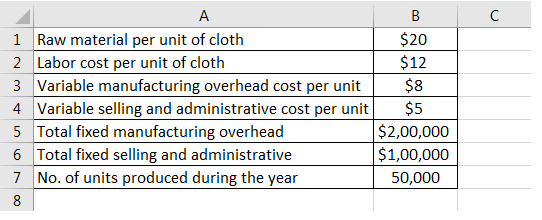It is to be noted that selling and administrative costs (both fixed and variable costs) are periodic costs in nature and as such are expensed in the period in which it occurred. However, these costs are not included in the calculation of product cost as per the AC.
Therefore, the calculation of AC is as follows,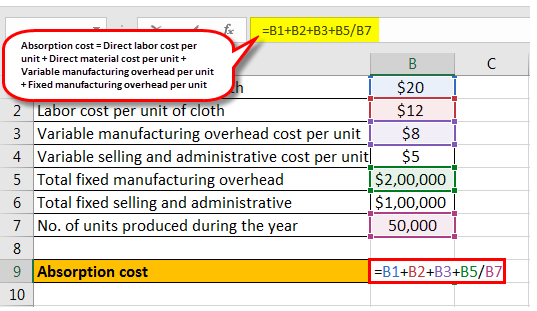Absorption cost Formula = Direct labor cost per unit + Direct material cost per unit + Variable manufacturing overhead cost per unit + Fixed manufacturing overhead per unit
= \$20 + \$12 + \$8 + \$200,000 / 50,000
AC will be –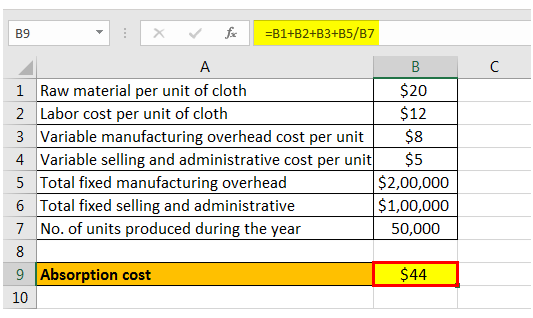Ab cost = \$44 per unit of cloth

Example 2.
Let us take the example of company ABC Ltd which is a manufacturer of mobile phone covers. Recently, the company has received an order for 2,500,000 mobile covers at a total contract price of \$5,000,000. However, the company is not sure whether the order is a profitable proposition. Do the calculation of Absorption Costing to find the order is profitable or not. The following are the excerpts from the entity’s income statement for the calendar year ending in December 2017: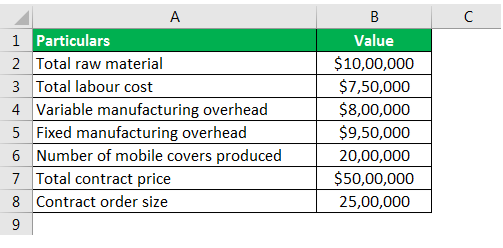Now, based on the above information, do the calculation of Absorption Costing.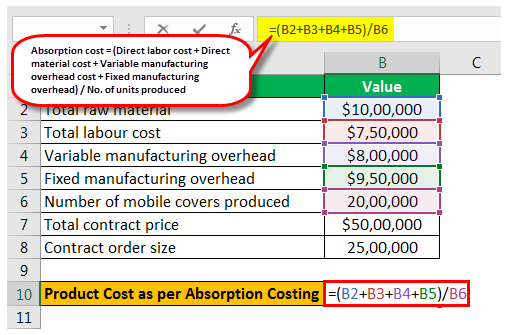Absorption cost formula = (Direct labor cost + Direct material cost + Variable manufacturing overhead cost + Fixed manufacturing overhead) / No. of units produced
AC = (\$1,000,000 + \$750,000 + \$800,000 + \$950,000) ÷ 2,000,000
AC will be –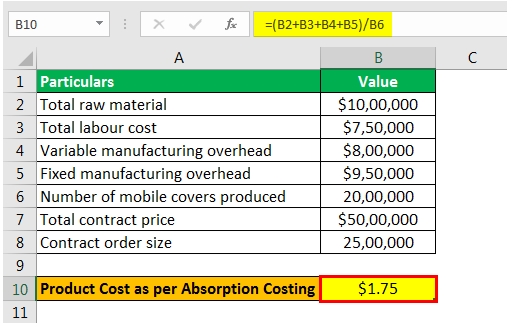AC = \$1.75 per mobile case
As per the contract pricing, the per unit price = \$5,000,000 / 2,500,000 = \$2.00 per mobile case
Since the formula of absorption costing method shows lower product cost than the pricing offered in the contract, the order should be accepted.

Relevance and Use of Absorption Costing Formula
It is very important to understand the concept of AC formula because it helps a company to determine the contribution margin of a product and that eventually helps in the break-even analysis. Based on the break-even analysis can decide the number of units required to be produced by the company in order to be able to book a profit. Further, the application of AC in the production of additional units eventually adds to the bottom line of the company in terms of profit since the additional units would not cost the company any additional fixed cost. Another advantage of AC is that it is GAAP compliant.

Offer running on EduRev: Apply code STAYHOME200 to get INR 200 off on our premium plan EduRev Infinity!

## Cost Accounting

131 videos|139 docs

,

,

,

,

,

,

,

,

,

,

,

,

,

,

,

,

,

,

,

,

,

;